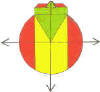DMD   EDU-HOME

WELCOME TO THIS PAGE INTENDED FOR STUDENTS AT THE UNIVERSITY OF THE WESTERN CAPE REGISTERED  FOR THE MODULE:

QSF  131

QSF   :   15 JANUARY 2010

In order to have reasonable prospects of success in your studies, you have to master basic quantitative skills in numbers, fractions, algebra and graphs. (cf p.1, QSF 131 Course Outline 2010)

HOW:

·                     PLAN OF ACTION TO TRANSFORM STUDENTS

·                     COURSE: LECTURES/TUTS/TESTS/EXAMS

·                     TAKES PLACE DURING FIRST OR SECOND SEMESTER

SHORT ACTIVITY

OBJECTIVE:         TO GET TO KNOW THE PERSON NEXT TO YOU  BETTER

‘QUANTITATIVELY’,   speaking.

TO GIVE YOU SOME IDEA OF THE QSF COURSE WORK

TO GET YOU ‘THINKING’

OUTCOME(S):     THIS IS BEST LEFT UNANSWERED! (IN EFFECT,

YOU  CAN WORK IT OUT YOURSELF, if it matters in your opinion!)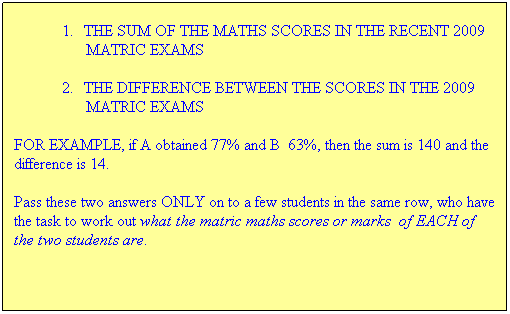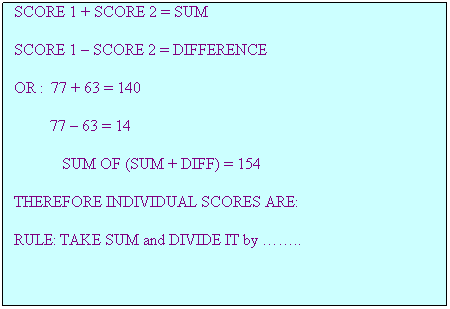************************

# University of the Western Cape

### Faculty of Economic and Management Sciences

B.COM. (GENERAL) Four-year Programme

## QSF 131

2010

In her famous diary which describes South African colonial life from 1797 to 1801, Lady Anne Barnard wrote the following on 1 June 1800:

“ … (The wife of ) Colonel Mercer… is…the longest woman I ever saw in my life…

Her Colonel is four inches taller than she ; they measure twelve feet seven inches together”

QUESTIONS:

(a)     How tall, in metres, is the couple altogether? (Use:1 inch = 2.54 cm; Ans : 3.8354 m)

(b)     Express the ratio of the two heights in its simplest form. (Ans :  147 : 155)

(c)     If the couple shared 20 koeksisters in the ratio of their heights, what % did each one have?

(d)     Why it is impossible to have the height of either the man or the woman in feet and inches which are both whole numbers?

(e)     Investigate how you could calculate the respective heights of each of the man and his wife, without using algebraic equations.

Anderson, H.J. South Africa a Century Ago: Letters and Journals (1797-1801) by  Lady Anne Barnard. Cape Town: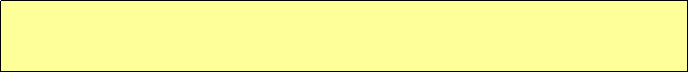BASIC QUESTION:

If an article costs R50 (VAT Excl) and the same article costs R57 (VAT Incl), determine the  % VAT.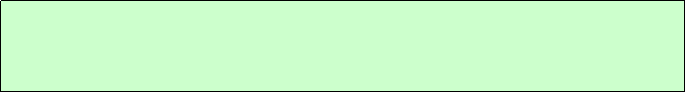OTHER QUESTIONS

1. An article costs R50 (Excl VAT). If VAT is 14%, calculate the cost of the article, inclusive of VAT.VAR 1:

If an article cost R57 (Incl. VAT of 14%), determine:

(a)   the VAT

(b)   the cost (Excl. Vat)

VAR II

If the VAT (at 14%) on an article is R7, determine:

(a)   the cost (Vat INCLUSIVE)

(b)   the cost (Vat EXCLUSIVE)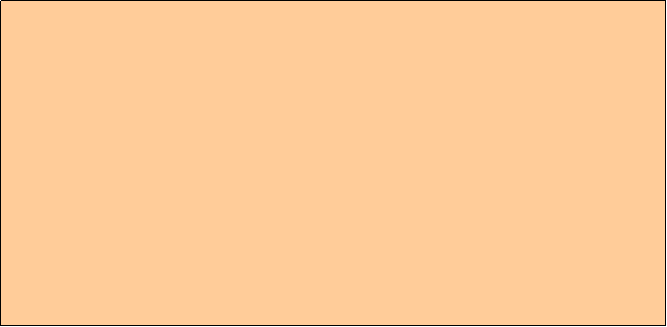FUNDAMENTAL PRINCIPLE:

DETERMINE THE FOLLOWING:

Which AMOUNT REPRESENTS 14%, 100% and 114%

Then use the method:

If ……(a) …..%   gives ………………..(the GIVEN Amount)

Then 1 % will be …………………. DIVIDE AMOUNT BY (a)

Then ………..% will be ……………..(the required amount)

REVISION PROBLEMS FOR QSB 195182: AUGUST 2008

REVISION PROBLEMS FOR QSB 195182

PROBLEM 1

In the recent 2008 Olympic games held in the Bird’s Nest stadium in Beijing, the Jamaican sprinter Usain Bolt ran the 100 metres in 9.69 seconds to clinch the gold medal for this event.

(a) Estimate his average speed over this distance in km per hour.

(b) Express his average speed over this distance in km per hour.

Solution

(a) One has a good idea of 20, 60 and 80 km/hour (stemming from our car driving experiences). Hence as good estimate would be 30-40 km/hr.

(b) Time needed to cover 100 metres  = 9.69 seconds

Since 1 km is ten times 100 metres, time taken to complete 1 km = 96.9 seconds = 1.615 minutes

(divide  by 60, since 60 secs = 1 minute)

Therefore, the distance covered in 1 hour = 60 divided by 1.615 = 37.15 km

Therefore Bolt’s average speed = 37.15 km/hour

PROBLEM 2

A special type of engine oil treatment is sold for R119 for 350 ml at a particular store. The same store also sells a 500 ml container of the same thing for R149. Which is the better buy?

Partial Solution:

Work out the respective prices per ml for each OR

Work out the respective quantities per rand.

PROBLEM 3

When laying a wooden floor of a room, a workman hammered in nails at an average rate of two nails every 2 minutes and 15 seconds. In total, 550 nails were needed to do the job.  If the average time taken to hammer in the nails becomes 0.125 seconds less each time after the first 10 had been hammered in, calculate the total time in hours taken to hammer in ALL the nails?

Solution:

Time to hammer in 10 nails = 2.25 × 5 = 11.25 minutes

Time to hammer in 11th nail = (½ of 2.25×60 – 0.125) secs = 67.375 secs  = a

d = 0.125 secs

n = 540

Thus           T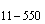=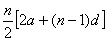=secs

=   18191.25 secs

=   5.053125 hours

=   5 hours 3 mins 11.25 secs

The following should help you improve your knowledge of the various formulae of the perimeter and area of certain well-known shapes.

TASK: CCFO: Identifying  Complete the following table. The first one has been done as an example.

 SHAPE DIAGRAM PERIMETER AREA/VOLUME FORMULA Rectangle l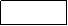b                     b                         l Perimeter 2l + 2b Rectangle lb                     b               l l x b Square Perimeter 4 x s Square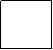s x s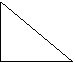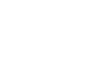Area ½ x Base x       height Parallelo-gram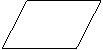Area Trapezium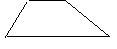½ x (sum of parallel sides) x height CircleArea Circle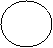Perimeterx Diameter P=2××radius Rhombus Area ½ × Product of the Diagonals

Area of rectangle = l x b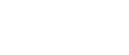Diagram for a square is:s

Area of Square = s x s

Area of Triangle = ½ x base x height. Area of Parallelogram = base x height

Area of Trapezium is = ½ x (sum of parallel sides) x height

Area of a Circle = (

Perimeter of a Circle = x D.Diagram of a rhombus is: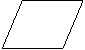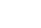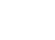Note:  A parallelogram can be completed from any triangle. Therefore, since the area of a parallelogram is base × height, the formula for the area of any triangle is equal to ½  of the area of a parallelogram with the same base and height.SHAPES: SURFACE AREA AND VOLUME

SHAPES IN 3-DIMENSIONS

3-Dimensional objects have length, breadth as well as height or depth. Their shapes consist of 2-dimensional figures like rectangles, squares and circles.

 DEFINITION   A right rectangular prism is one where all the side and end planes are perpendicular to each other.  An example of this figure is a room where the walls are all rectangles and are at right angles to the floor and the roof.   A cube is a right rectangular prism with all the sides equal.   A right cylinder has circular ends and a curved surface perpendicular to these ends. A good example of a right cylinder is a tin can.   A sphere is the shape which a circle maps out if rotated about it’s a central diameter or axis. A tennis ball is an example of a sphere.

These shapes have an ‘outside’ or surface area. They also all have ‘inner’ size or volume.

SURFACE AREA

Surface area is still an ‘area’ and is also measured in square units.

In order to get a practical idea of the surface area of a right cylinder, we give you the following task:

 ACTIVITY CCFO: Identifying and Collecting Make a list of objects in your home which represent different shapes. Find an empty toilet roll, which you then cut open to form a rectangle. Determine the area of this rectangle and the height. What was the diameter of the circle? Answers: 1. Shapes: Rectangles: books, table tops, windows, etc.    Cylinders: cups, glasses 3. Area = length × breadth 4. The breadth of rectangle when the toilet role was cut open.

This curved area of any right cylinder such as the toilet roll, is called the curved surface area. This area can be found by multiplying the perimeter of the circular base by the height. Thus the curved surface area = π × D × height.

VOLUME OF A RIGHT RECTANGULAR PRISM

Volume is measured in cubic units. We use S.I. units such as m³, cm³ or mm³ to the volume of a 3-dimensional object.

Similar to how we determined the area of a rectangle, we may use cubes of 1 cm by 1cm by 1cm (or 1 cm³) to determine the volume of a right rectangular .

©  DESMOND DESAI, DMD EDU-HOME, 2008

All rights reserved. Designed and created by Desmond Desai, South Africa. This page is protected by Copyright. No part of this page may be reproduced, stored in a retrieval system, or transmitted in any form of by any means, electronic, mechanical, photocopying, recording or otherwise, without the prior written permission of the copyright holder.